Courses

# Newton's Third Law of Motion Class 9 Notes | EduRev

## Class 9 : Newton's Third Law of Motion Class 9 Notes | EduRev

The document Newton's Third Law of Motion Class 9 Notes | EduRev is a part of the Class 9 Course Science Class 9.
All you need of Class 9 at this link: Class 9

Newton's third law of motion
Definition : For each and every action, there is equal and opposite reaction.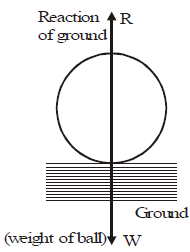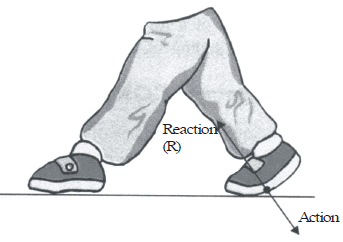lf a body A exerts force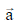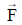on another body B, then B exerts a force -on A, the two forces acting along the same line. The two forcesand -connected by Newton's third law are called action-reaction pair. Any one may be called 'action' and the other 'reaction'.

Action and reaction acts on different bodies hence they never cancel each other.

Note : Action and reactions forces occur simultaneously. It is wrong to think that first action occurs and it is followed by reaction.

An important conclusion :- When action-reaction forces are acting on two bodies of masses mA and mB then
From eqn. FAB = -BA
mAaA = -mBaB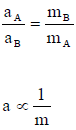Misconcept : The action and reaction forces acting on a body are equal and opposite and therefore cancel out the effect of each other.
Concept : Action and reaction do not cancel out the effect of each other because they act on different bodies.
Misconcept : Any pair of equal and opposite forces is a action-reaction pair.
Concept : Only those forces from an action-reaction pair which involve the two bodies responsible for action-reaction.

Some other examples of Newton's third law :
1. When a bullet is fired from a gun, we observe recoiling of the gun. The gun acts on bullet i.e., exerts a force on the bullet; simultaneously, the bullet reacts on the gun.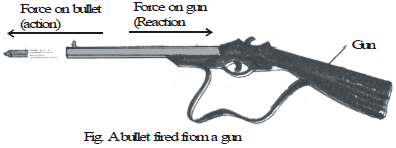2. While walking or running, you push the ground in the backward direction with your feet. The ground simultaneously exerts a force of equal magnified in the forward direction on feet. This force enables us to walk.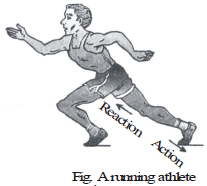3. When a man jumps from a boat, the boat also experiences a backward jerk. This is due to the action-reaction pair as shown in figure.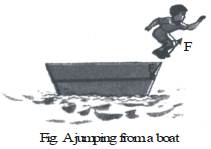4. Inflate a balloon and leave it. You will observe that the balloon moves in opposite direction to the opening in balloon through which the air is coming out.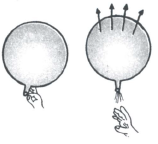5. A ball rebounds after striking against a floor.
When a ball strikes against a floor, it exerts a force on the floor. According to Newton's third law of motion, the floor exerts an equal and opposite force on the ball. Due to this reaction, the ball rebounds.

6. How do we swim ?
While swimming, a swimmer pushes the water backward with his hands. The reaction offered by the water to the swimmer pushes him forward.

7. A boatman pushes the river bank with a bamboo pole to take his boat into the river.
When the boatman pushes the river bank with a bamboo pole, the river bank offers an equal and opposite reaction. This reaction helps the boat to move into the river.

8. Why a fireman struggles to hold a hose-pipe ?
A fireman has to make a great effort to hold a hose-pipe to throw a stream of water on fire to extinguish it. This is because the stream of water rushing through the hose-pipe in the forward direction with a large speed exerts a large force on the hose-pipe in the backward direction.

Law of conservation of momentum
We know that if a moving body strikes a body at rest, the moving body slows down and the stationary body starts moving. Whereas the first body loses momentum, the second body gains momentum. We shall observe that the total momentum before impact is equal to total momentum after impact.

If two bodies of masses m1, m2 are initially moving with velocities u1, u2 and after collision they start moving with velocities vI and v2 respectively, then

m1u1 + m2u2 = m1v1 + m2v2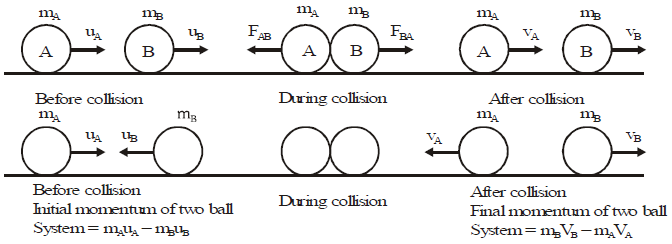i.e., total momentum before collision is equal to total momentum after impact.

If one body gains momentum, the other loses an equal amount of momentum.

Proof of the law of conservation of momentum
Consider a system consisting of two bodies A and B of (say glass spheres) masses m1 and m2 respectively. Let these bodies are moving with velocities u1 and u2 as shown in figure. Let u1 > u2. Let these bodies collide with each other for a small interval of time 'dt'. At the time of collision, body A exerts a force F on body B and body B exerts equal and opposite force ( F) on body A. Due to these forces, the momentum of the bodies changes. Let n1 and n2 be the velocities of body A and body B respectively after the collision.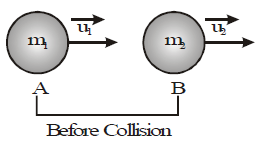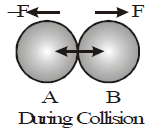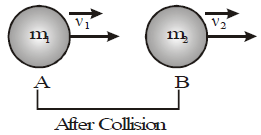Initial momentum of body A = m1 u1
Initial momentum of body B = m2 u2
Final momentum of body A = m1 n1
Final momentum of body B = m2 v2
Total momentum of the system before collision = m1u1 + m2u2
Total momentum of the system after collision = m1u1 + m2u2
Now, the rate of change of momentum of body A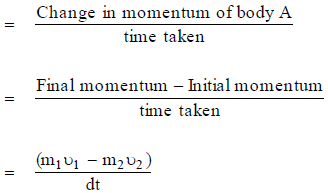According to Newton's second law of motion,
Force acting on body A = rate of change of momentum of body A

i.e.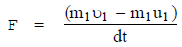... (1)
According to Newton's second law of motion force acting on body B = rate of change of momentum of body BNow, rate of change of momentum of body B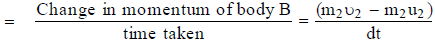i.e.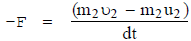or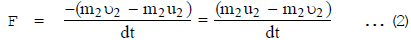Equating eqns. (1) and (2), we get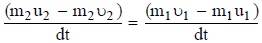or m1u1 + m2u2 = m1u1 + m2u2

i.e. Total momentum of the system (i.e. bodies A and B) before collision
= Total momentum of the system (i.e. bodies A and B) after collision.
This is the law of conservation of linear momentum.

Examples to illustrate the law of conservation of momentum
1. Recoil of a gun. The recoil of a gun can be explained with the help of the law of conservation of momentum.
The bullet inside the gun and the gun itself form a system. Thus, the system is gun bullet. Before firing, the gun and the bullet are at rest, therefore, momentum of the system before firing is zero.
When the bullet is fired, it leaves the gun in the forward direction with certain momentum. Since no external force acts on the system, so the momentum of the system (gun bullet) must be zero after firing. This is possible only if the gun moves backward with a momentum equal to the momentum of the bullet. That is, why gun recoils or moves backward figure.

Recoil Velocity. The velocity with which the gun moves backward after firing a bullet is known as recoil velocity.

Calculation of Recoil Velocity of a Gun
Let mass of the bullet = m
Velocity of the bullet after firing =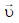Mass of the gun = M
Velocity of the gun after firing =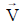Since the system is at rest before firing, so momentum of the system (gun bullet) before firing = 0
Total momentum of the system (gun bullet) after firing
= momentum of gun + momentum of bullet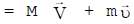According to the law of conservation of momentum
Momentum of the system after firing = Momentum of the system before firing

i.e.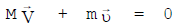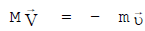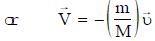Negative sign shows that the direction of the velocity of the gun after firing is opposite to the direction of the velocity of the bullet.

2. Rocket propulsion (Movement of a rocket in the upward direction)
The movement of a rocket in the upward direction can also be explained with the help of the law of conservation of momentum.
The momentum of a rocket before it is fired is zero. When the rocket is fired, gases are produced in the combustion chamber of the rocket due to the burning of fuel. These gases come out of the rear of the rocket with high speed. The direction of the momentum of the gases coming out of the rocket is in the downward direction. To conserve the momentum of the system (rocket gases), the rocket moves upward with a momentum equal to the momentum of the gases. The rocket continues to move upward as long as the gases are ejected out of the rocket.

3. Inflated balloon lying on the surface of a floor moves forward when pierced with a pin.
The momentum of the inflated balloon before it is pierced with a pin is zero. When it is pierced with a pin. air in it comes out with a speed in the backward direction. To conserve the momentum, the balloon moves in the forward direction.
Newton's second law states that the force is proportional to the product of mass and acceleration. Second definition of 2nd law. The rate of change of momentum is proportional to the impressed force and takes place in the direction of force.
Force applied on a body is 1 N if it produces on a mass of 1 kg an acceleration of 1 m s-2.
Force applied on a body is 1 dyn if it produces on a mass of 1 g an acceleration of 1 cm s-2.
1 N = 105 dyn.

Momentum is the quantity of motion contained in a body and is equal to the product of mass and velocity. It is measured in kg m s-1 in SI and in g cms-1 in CGS.
Impulse is the product of force and time and is also equal to the change in momentum.
Its SI unit is Ns or kg m s-1 in CGS, it is measured in dyn s or g cm s-1

IMPORTANT POINTS TO REMEMBER

• Unit of force is dyn or newton whereas that of mass is gram or kilogram.
• It is wrong to say "Weight of Ankit is 40 kg." We should say "Mass of Ankit is 40 kg" or "Weight of Ankit is 40 × 9.8 = 392N."
• 1 metric ton = 1,000 kg , 1 quintal = 100 kg
• 1 metric ton = 10 quintals , 1 kg = 1,000 g
 Competitive Window  1. In solving the numericals, always take the force in absolute units i.e., in dynes or newtons. 2. 1 N = 105 dyn. 3. The kg f (kilogram force) and kg wt (kilogram weight) are two names of same quantity i.e., of practical units of force. 4. Momentum is a vector quantity. 5. Weight of the body or force applied should be never states in kg. Kilogram is a unit of mass and not of weight or force. Never say, "My weight is 50 kg". Say "My weight is 50 kg for 50 kg wt or 50 × 9.8 N" or say, "My mass is 50 kg". 6. Hold 0.1 kg on your hand placed parallel to earth. Your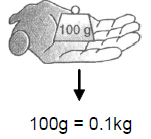hand is applying a force of nearly 1 N (0.98 N to be exact). 7. In all numericals, use only absolute units (N or dyn). If the force is given in kg f or g f, convert these to N or dyn.

Offer running on EduRev: Apply code STAYHOME200 to get INR 200 off on our premium plan EduRev Infinity!

## Science Class 9

143 videos|224 docs|135 tests

,

,

,

,

,

,

,

,

,

,

,

,

,

,

,

,

,

,

,

,

,

;